# Sine Function

The word sine comes from the Latin word sinus that literally translates to 'bay' or 'fold'. This in turn is either derived from a term that means bowstring.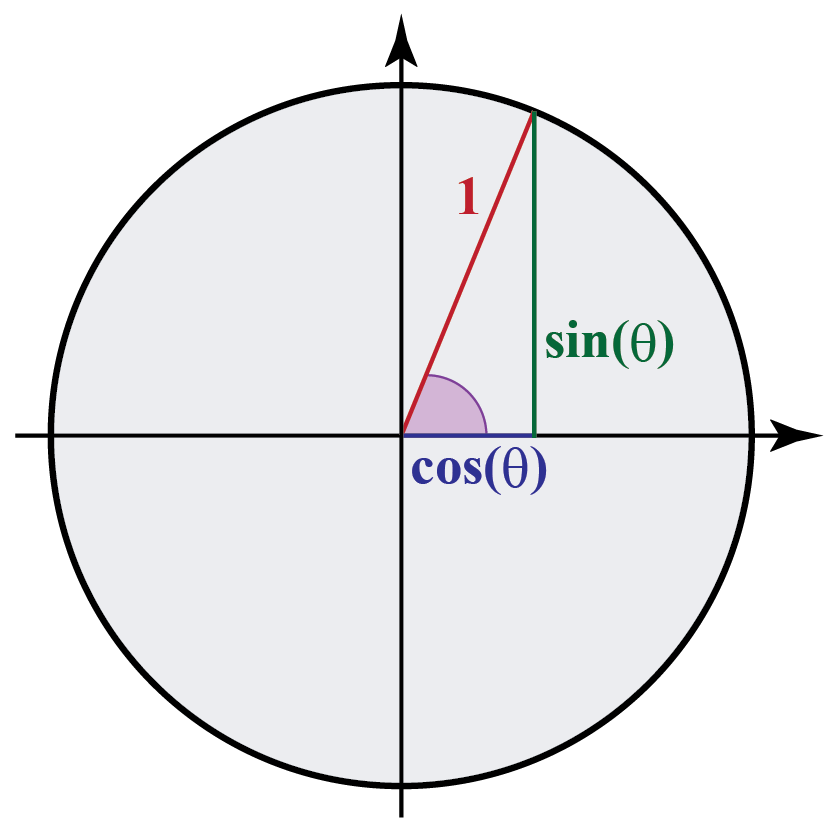In this mini-lesson, we will explore the world of the sine function. The journey will take us through sine function, unit circle, and angles, how to use them, and their various applications.

You can check out the interactive calculator to know more about the lesson and try your hand at solving a few interesting practice questions at the end of the page.

## Lesson Plan

 1 What Is a Sine Function? 2 Important Notes on Sine Function 3 Thinking out of the Box! 4 Solved Examples on Sine Function 5 Interactive Questions on Sine Function

## What Is a Sine Function?

The sine function is a periodic function in trigonometry.

The sine function can be defined as the ratio of the length of the perpendicular to that of the length of the hypotenuse in a right-angled triangle.

Let us try to understand the concept of sine function by analyzing the four quadrants of the coordinate axis system.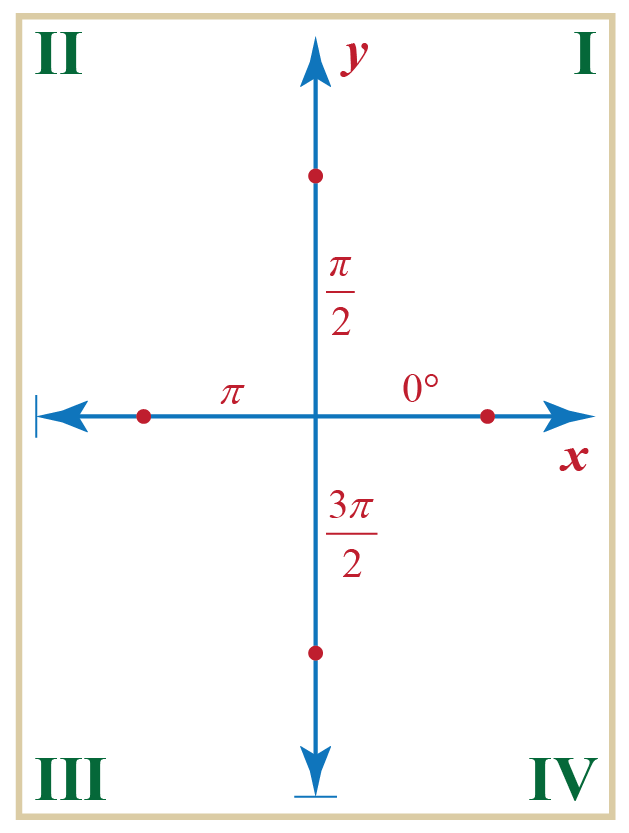Now, consider a unit circle centered at the origin of the coordinate plane.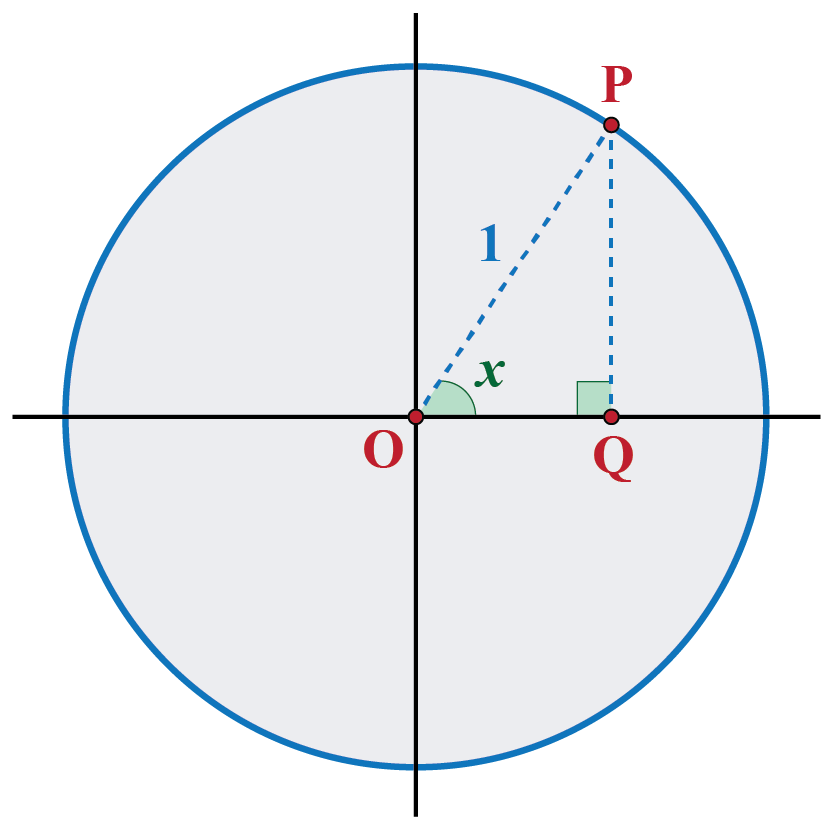A variable point $$P$$ moves on the circumference of this circle.

From the figure, we observe that $$P$$ is in the first quadrant, and $$OP$$ makes an acute angle of $$x$$ radians with the positive $$x$$-axis.

$$PQ$$ is the perpendicular dropped from $$P$$ onto the horizontal axis. The triangle is thus formed by joining the points O, P, and Q as shown in the figure.

Hence, the sine function for the above case can be mathematically written as:

 $$\sin x = \frac{{PQ}}{{OP}}$$

Here, x is the acute angle formed between the hypotenuse and the base of a right-angled triangle.

## Sine Graph

The following figure shows a unit circle with center $$O$$ at the origin, and a point $$P$$ moving along the circumference of this circle.

The angle which $$OP$$ makes with the positive direction of the $$x$$-axis is $$x$$ (radians).

$$PQ$$ is the perpendicular dropped from $$P$$ to the horizontal axis. We get a $$\triangle OPQ$$.

We note that: $\sin x = \frac{{PQ}}{{OP}} = \frac{{PQ}}{1} = PQ$

As $$x$$ varies, we study that:

The value of $$\sin x$$ varies with the variation of the length of $$PQ$$.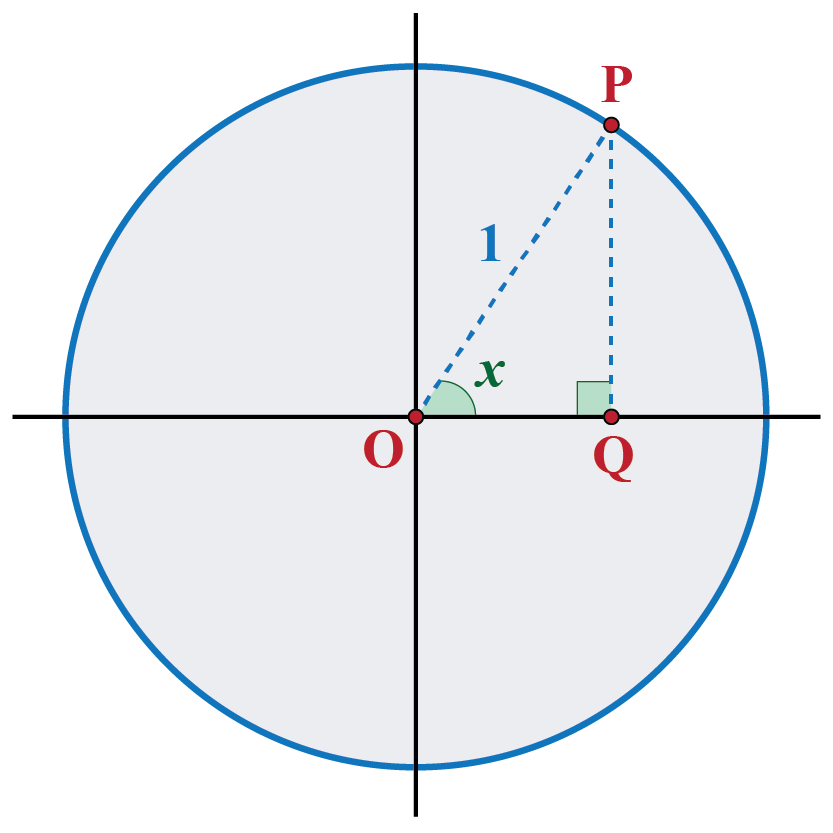Case 1: Variation of PQ in the first quadrant.

Suppose that initially, $$P$$ is on the horizontal axis. Let us consider a movement of $$P$$ through $$90^\circ$$ or $$\frac{\pi }{2}$$ rad.

The following figure shows different positions of $$P$$ for this movement: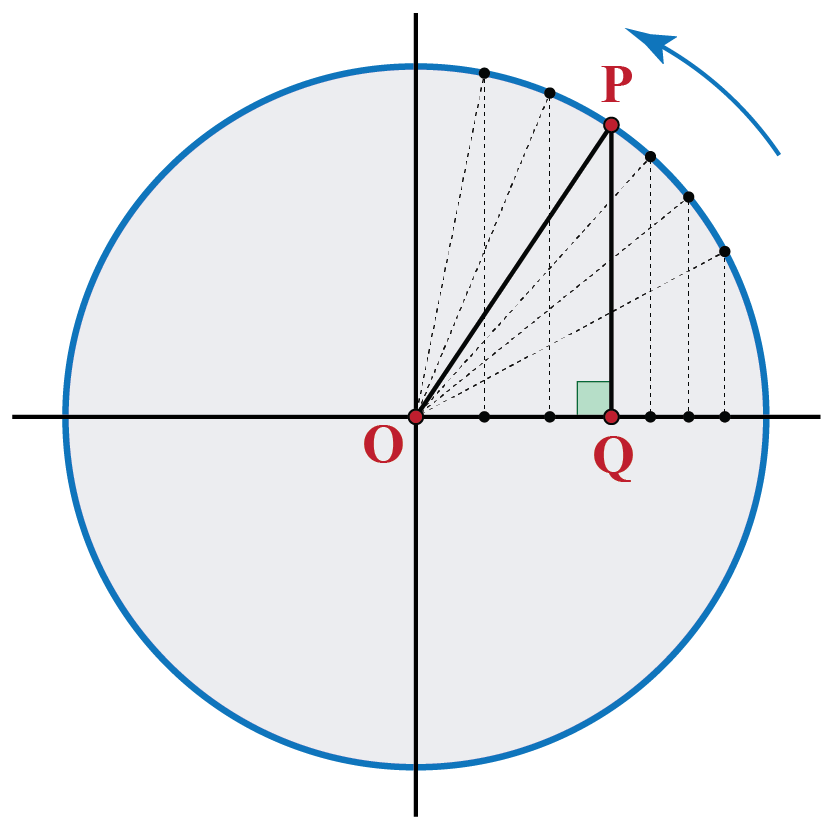Clearly, $$PQ$$ has increased in length, from an initial value of 0 (when $$x$$ is 0 radians) to a final value of 1 (when $$x$$ is $$\frac{\pi }{2}$$ radians).

We can now plot this variation.

The horizontal axis represents the input variable $$x$$ as the angle in radians, and the vertical axis represents the value of the sine function for $$x$$.

The plot thus obtained is shown below: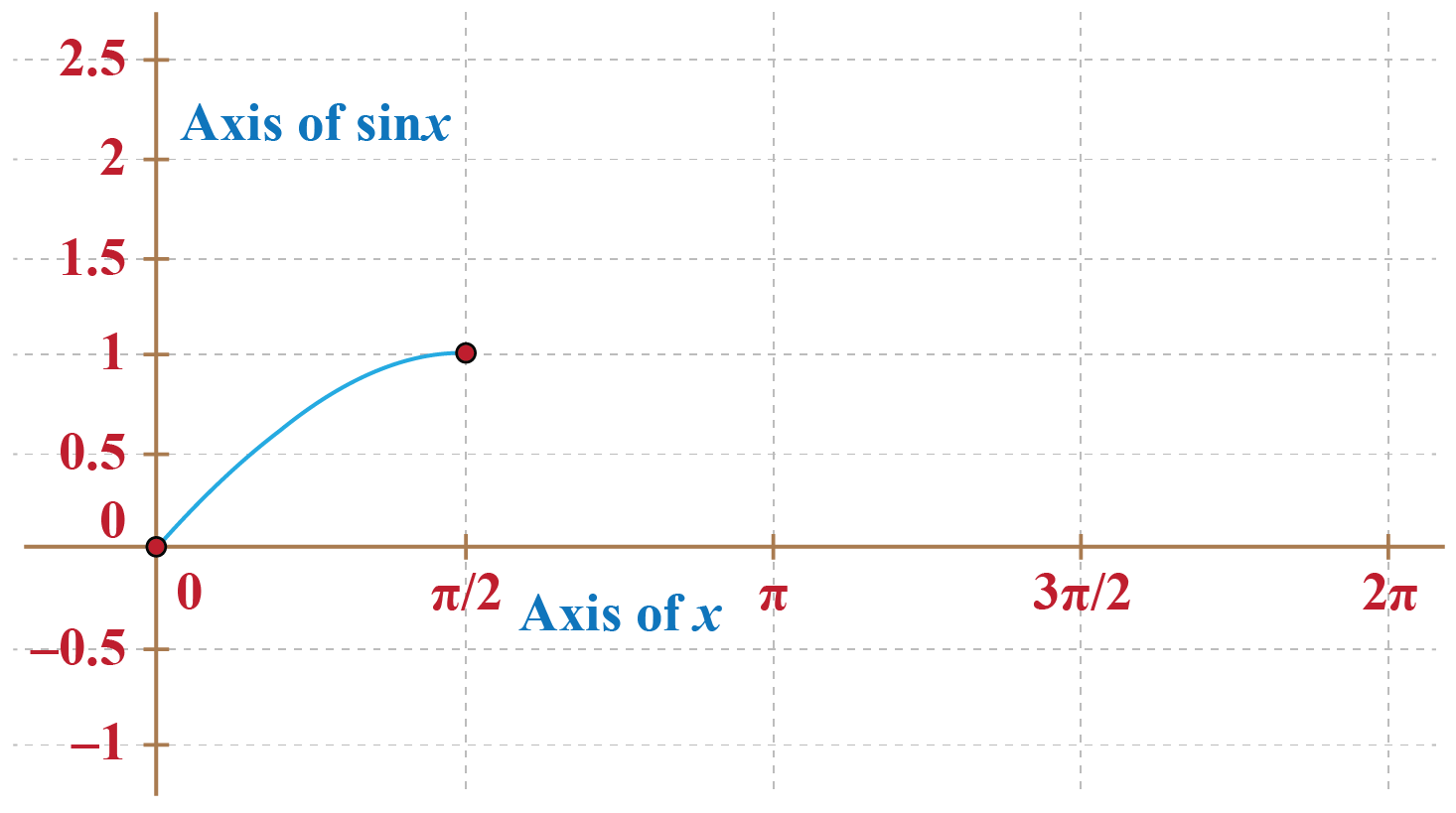Case 2: Variation of PQ in the second quadrant.

Now, let us see what happens as $$P$$ moves further.

The following figure shows different positions of $$P$$ as it subsequently moves from a $$90^\circ$$ position to $$180^\circ$$ position: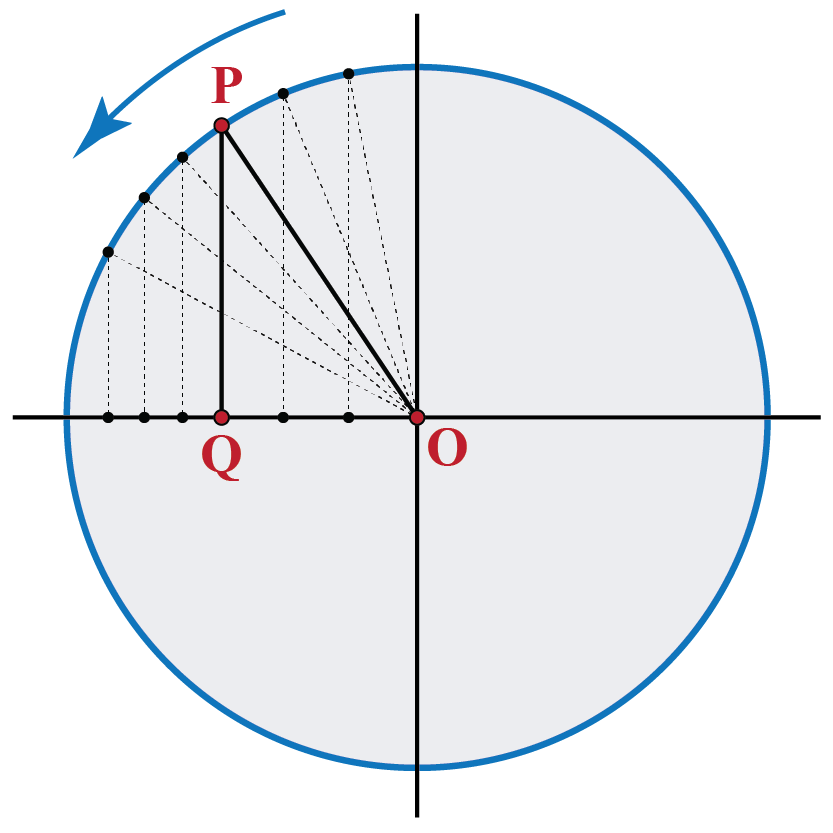In this phase of the movement, the length $$PQ$$ decreases, from a maximum of 1 at $$90^\circ$$, to a minimum of 0 at $$180^\circ$$.

We continue plotting this variation on the same graph we plotted earlier: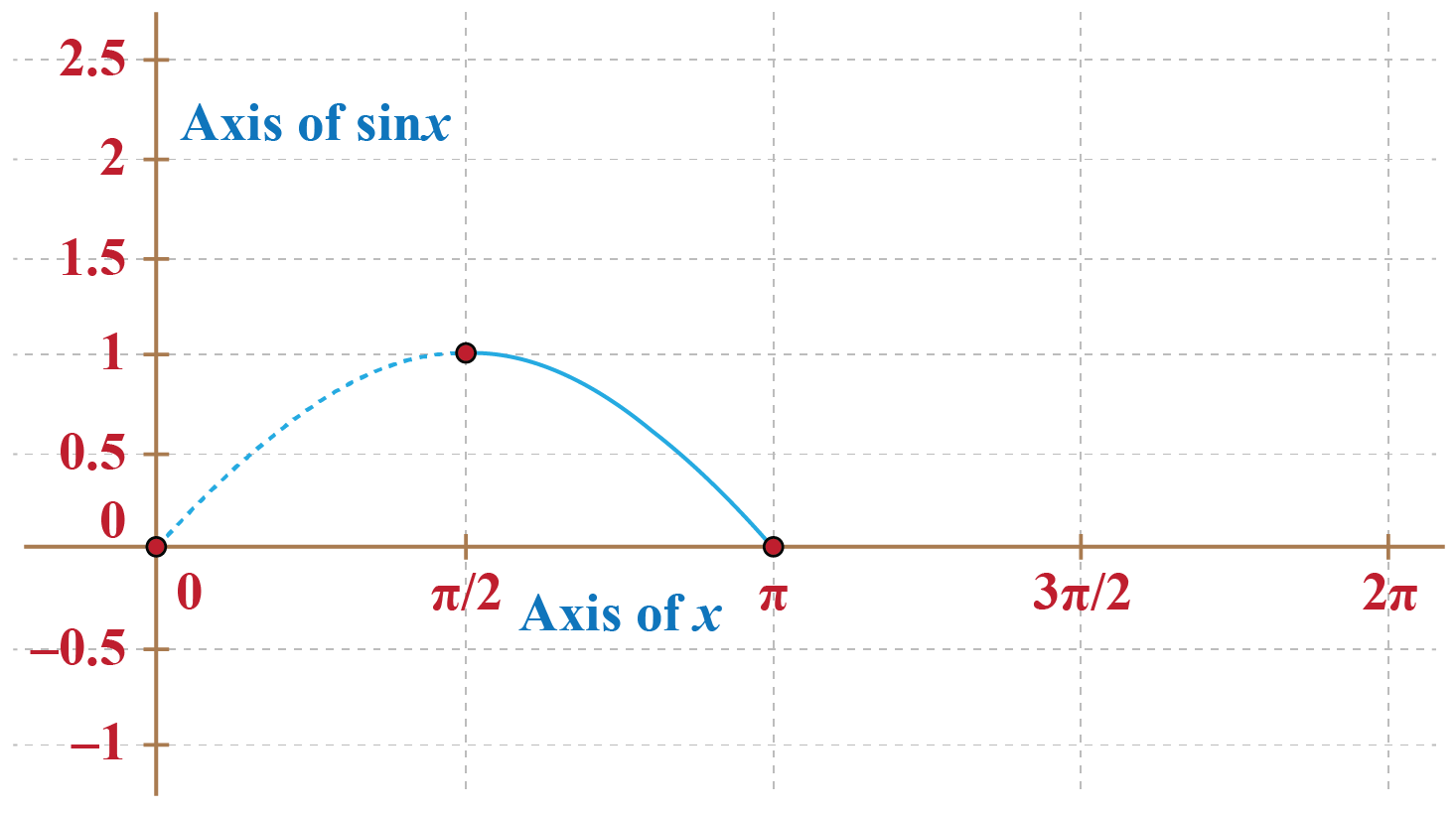Case 3: Variation of PQ in the third quadrant.

When $$P$$ moves from a position of $$180^\circ$$ to a position of $$270^\circ$$, though the length or magnitude of $$PQ$$ increases.

But since the direction is along the negative y-axis, the actual value PQ decreases from 0 to - 1.

Thus, the value of the sine function for angle x decreases.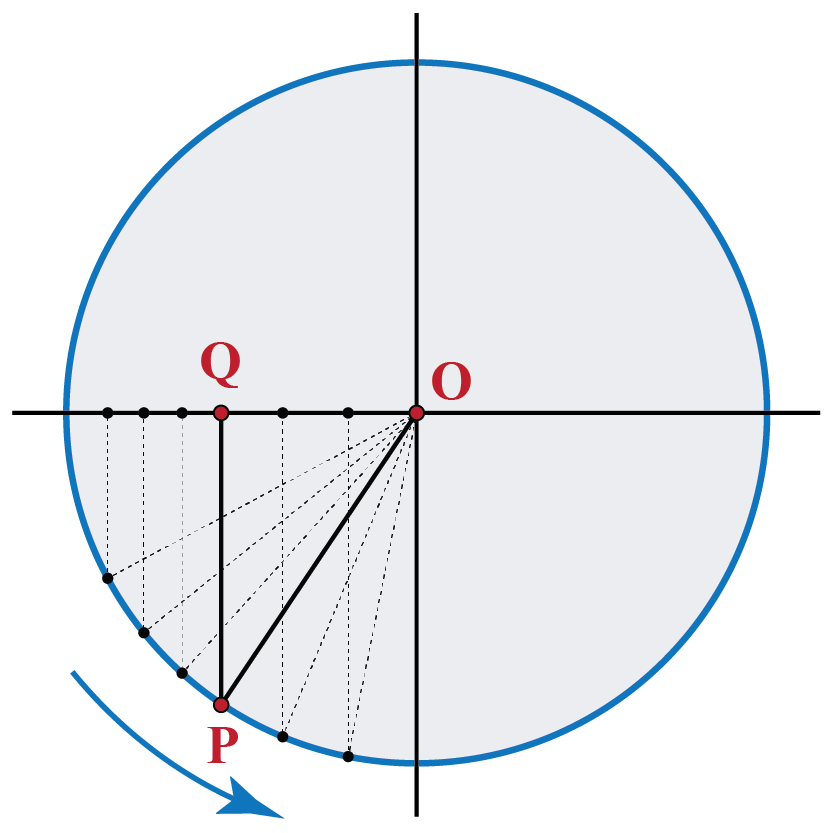We add this variation to our graph: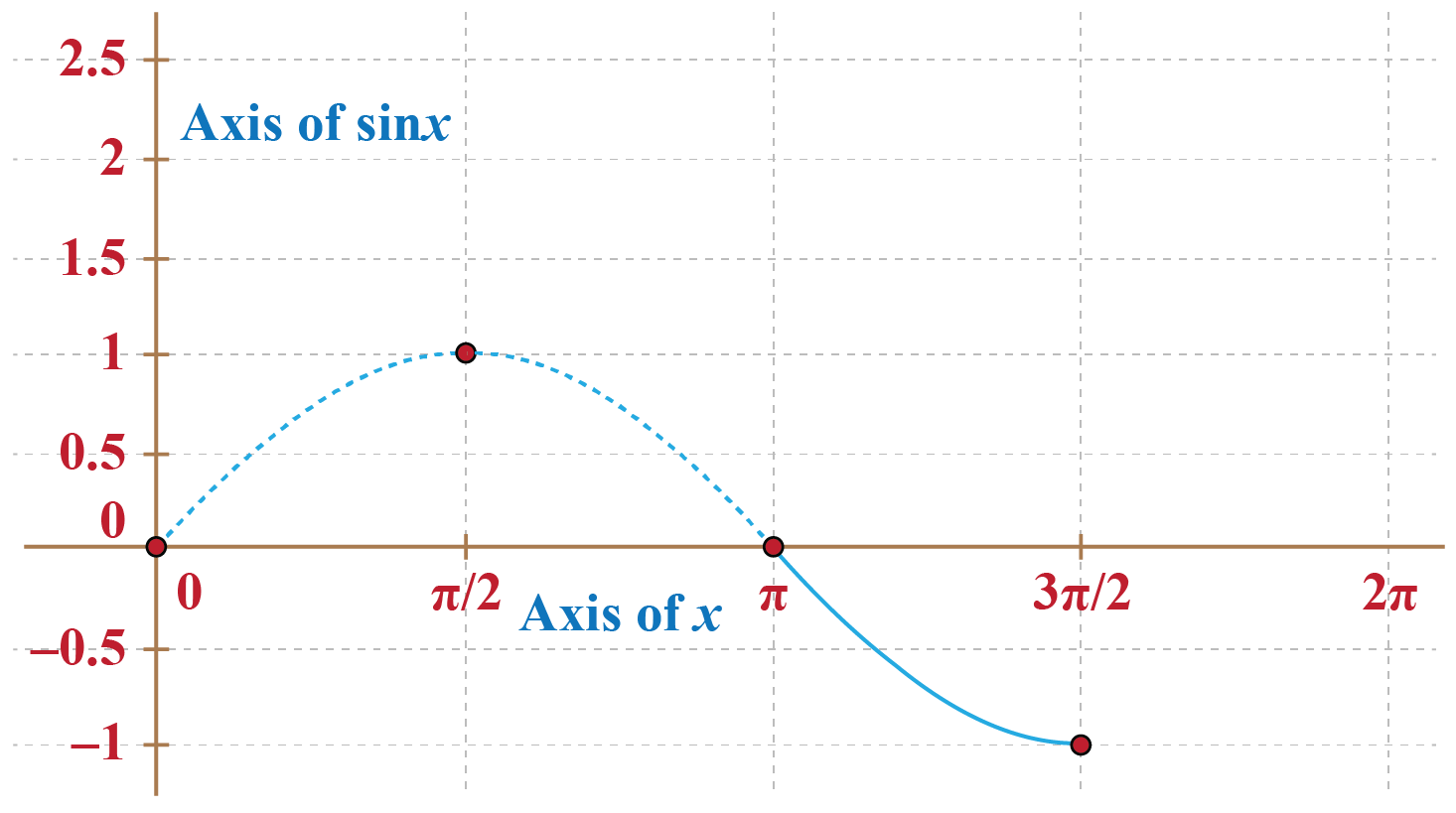Case 4: Variation of PQ in the fourth quadrant.

Finally, when $$P$$ moves from a position of $$180^\circ$$ to a position of $$360^\circ$$, $$PQ$$ increases from $$- 1$$ to 0 (once again.

Though the length or magnitude of $$PQ$$ decreases but the algebraic value of $$PQ$$will increase due to its direction is along the negative y-axis.

Thus, the value of the sine function for angle x increases.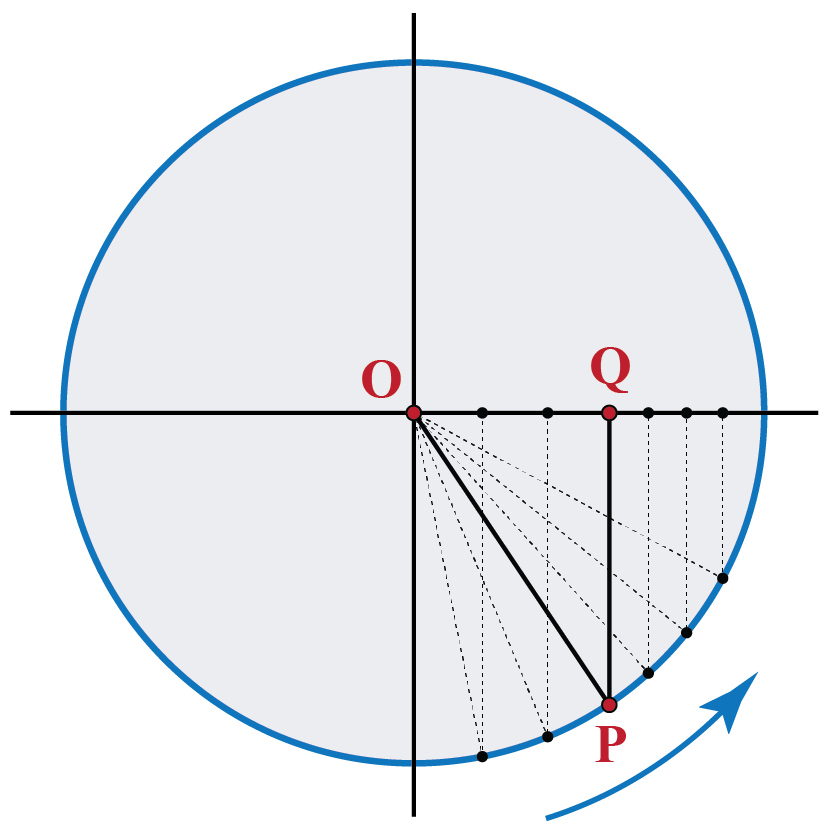Merging the response of variation in the value of $$PQ$$ for all four quadrants, we obtained the complete plot of PQ vs x or sin x vs x, for one complete cycle of $$0$$ radians to $$2 \pi$$ radians$$(0^\circ to 360^\circ)$$.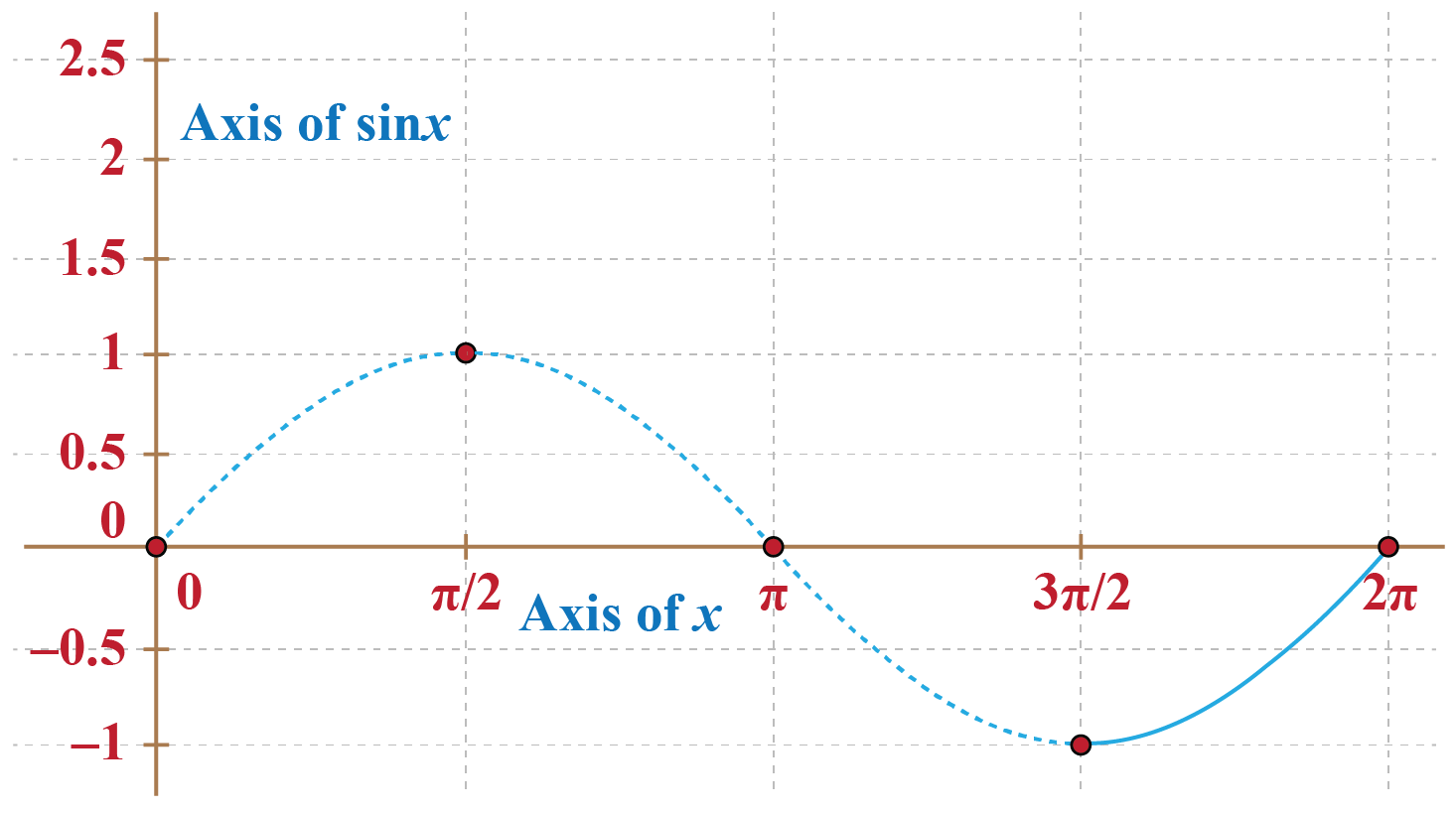What happens when $$P$$ moves further now? The same variation cycle starts all over again.

Thus, if we extend the sine function to take on all real inputs, we obtain the following graph: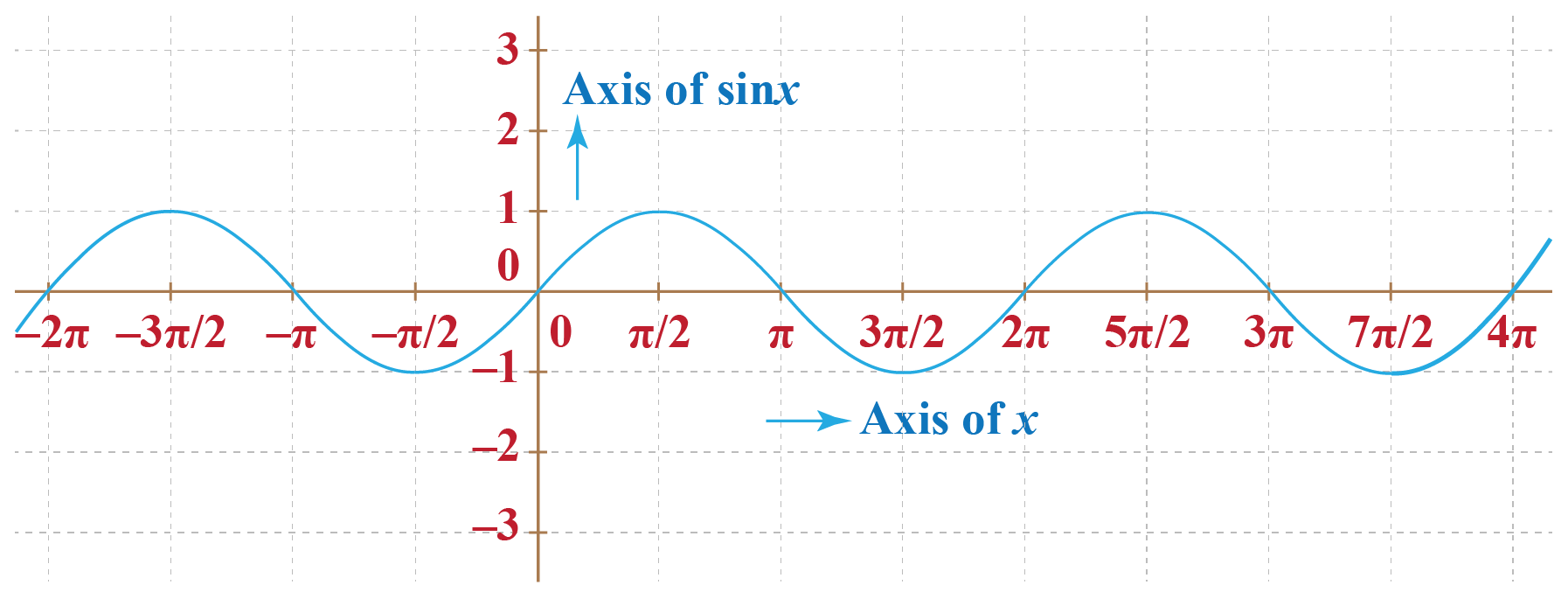## Basic Properties of Sine Function

Properties of sine function depend upon the quadrant in which the angle lies.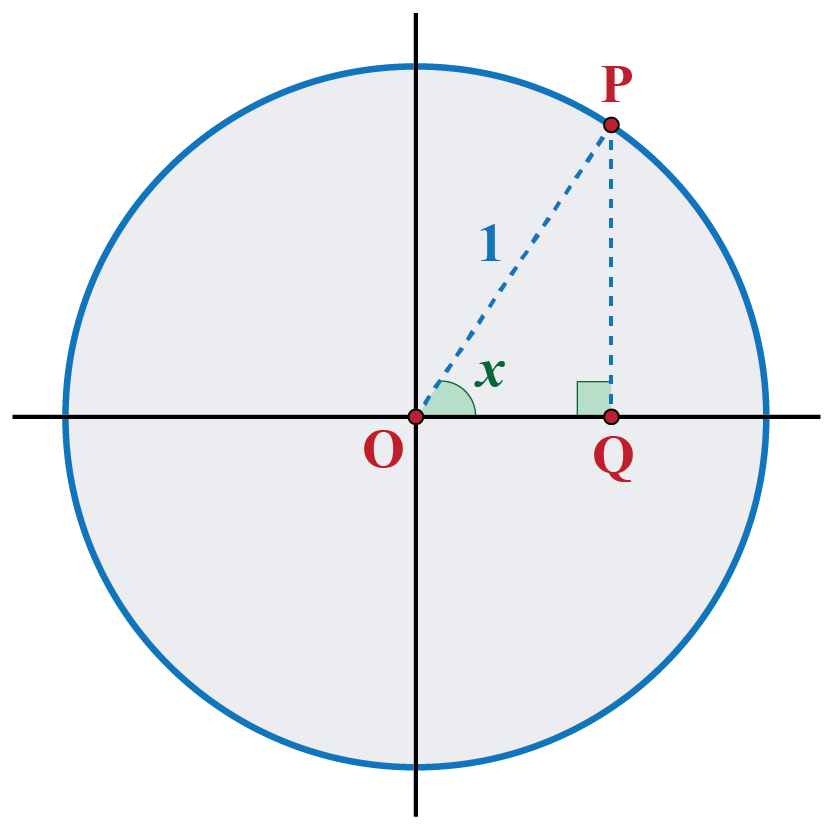From the above graph and circle relation, we can see that value of the sine function is positive in the first and second quadrant whereas, in the third and fourth quadrant, it bears a negative value.

This can also be observed for a few values of the sine function at different quadrants as shown in the table below:

 Sine Degrees Sine Values Sine 0° 0 Sine 30° 1/2 Sine 45° 1/√2 Sine 60° √3/2 Sine 90° 1 Sine 120° √3/2 Sine 150° 1/2 Sine 180° 0 Sine 270° -1 Sine 360° 0

## Range, Domain of Sine and Other Properties

Range and Domain of Sine Function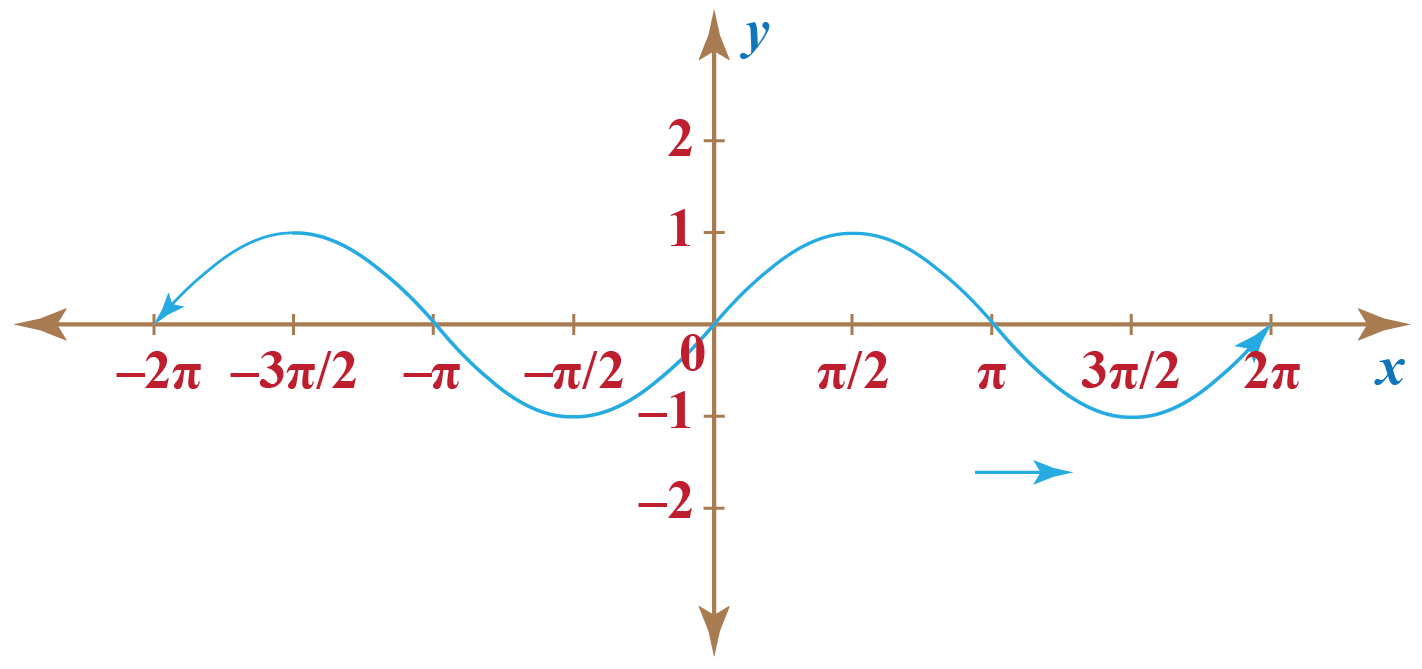Looking at the sine function on a domain centered at the y-axis, helps us bring out its symmetry. Thus, we can see in the figure above, the sine function is symmetric about the origin.

So the domain of sine is all real numbers. The range of sine is [-1,1].

Domain of Sine: R

Range of Sine: [-1,1]

Properties of Sine Functions

• The sine function is a periodic function
A periodic function is a function which when has a specific horizontal shift, P, results in a function equal to the original function, i.e.,
$f(x + P) = f(x)$
for all values of x within the domain of f.

The sine graph repeats itself after 2π, which suggests the function is periodic with a period of 2π.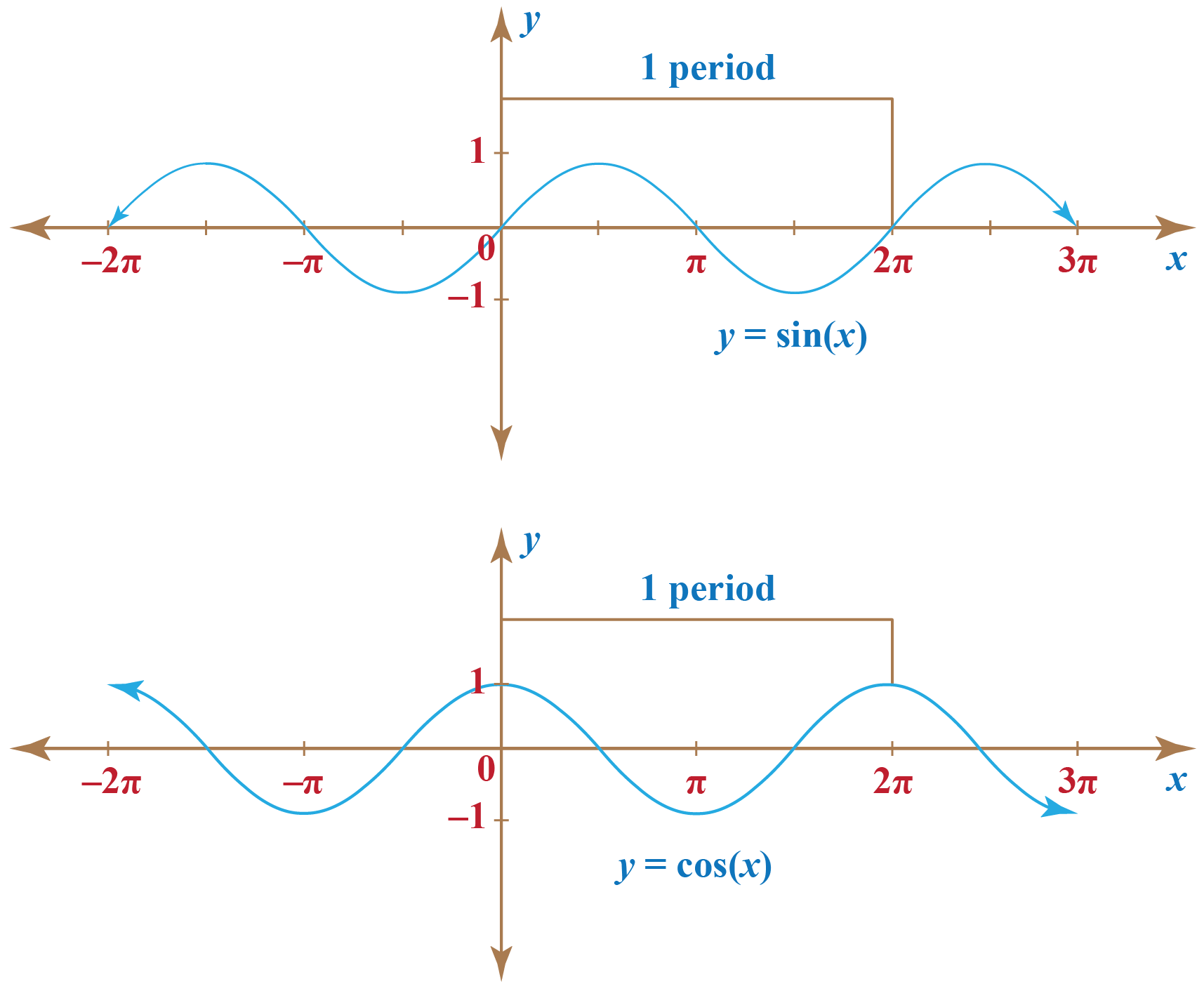So we can say that:

$\sin \left( {x + 2n\pi } \right) = \sin x\,\,\,{\rm{for \,\,\,every}}\,x$

For example,

\begin{align}&\sin \left( {1000\pi + \frac{\pi }{3}} \right) = \sin \frac{\pi }{3}\\&\sin \left( {\frac{{2\pi }}{3} - 128\pi } \right) = \sin \frac{{2\pi }}{3}\end{align}

• The sine function is an odd function:

The sine function is an odd function because $\sin(−x) = −\sin x.$

## Solved Examples

 Example 1

Find the values of

a) $$\sin {780^0}$$

b) $$\cos \left( { - {{660}^0}} \right)$$

c) $$\tan \left( {\frac{{41\pi }}{4}} \right)$$

d) $$\cot \left( { - \frac{{19\pi }}{3}} \right)$$

Solution

(a) We have:

\begin{align}{780^0} &= {720^0} + {60^0}\,\,\, \\\Rightarrow \,\,\,{780^0} &\equiv {60^0}\\ \Rightarrow \,\,\,\sin \left( {{{780}^0}} \right) &= \sin \left( {{{60}^0}} \right) \\&= \frac{{\sqrt 3 }}{2}\end{align}

 $$\therefore \frac{{\sqrt 3 }}{2}$$

(b) We note that:

\begin{align}- {660^0} &= - {720^0} + {60^0}\\ \Rightarrow - {660^0} &\equiv {60^0}\\ \Rightarrow \cos \left( { - {{660}^0}} \right) &= \cos \left( {{{60}^0}} \right)\\ &= \frac{1}{2}\end{align}

 $$\therefore \frac{1}{2}$$

(c) We have:

\begin{align*} \frac{{41\pi }}{4} &= 10\pi + \frac{\pi }{4}\,\,\, \\ \Rightarrow \frac{{41\pi }}{4} &\equiv \frac{\pi }{4} \\ \Rightarrow \tan \left( {\frac{{41\pi }}{4}} \right) &= \tan \frac{\pi }{4} \\ &= 1 \end{align*}

 $$\therefore\tan \left( {\frac{{41\pi }}{4}} \right) = 1$$

(d) We have:

\begin{align} - \frac{{19\pi }}{3} &= - 6\pi - \frac{\pi }{3}\,\,\, \\ \Rightarrow - \frac{{19\pi }}{3} &\equiv - \frac{\pi }{3} \\ \Rightarrow \cot \left( { - \frac{{19\pi }}{3}} \right) &= \cot \left( { - \frac{\pi }{3}} \right) \\ &= - \sqrt 3 \end{align}

 $$\therefore - \sqrt 3$$
 Example 2

Daniel wants to find the value of x for the given $$\triangle$$MNO if it is known that $$\sin \theta = 0.6$$. Can you help him in solving this?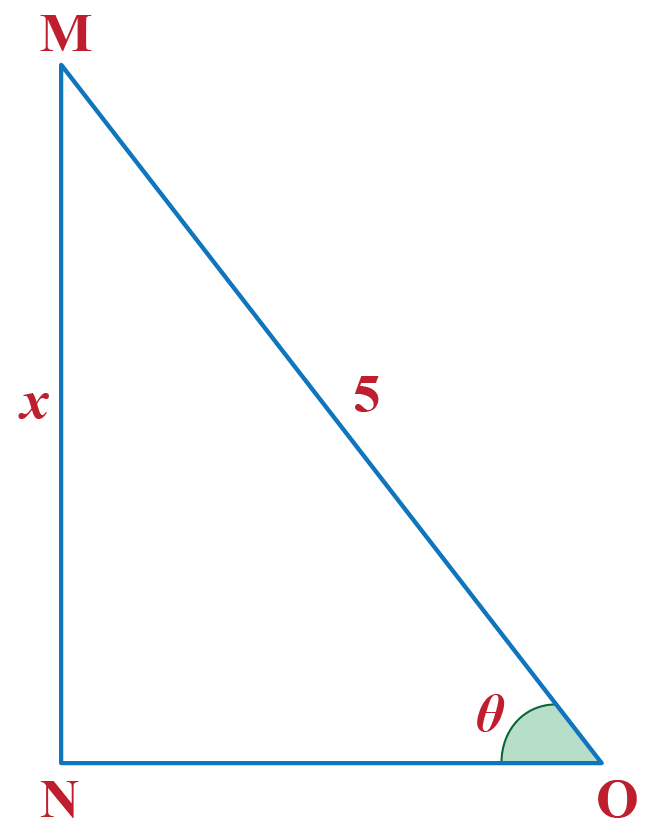Solution

Given,   $\sin \theta = 0.6$

Since,\begin{align*} \sin \theta &=\dfrac{\text {Perpendicular}}{\text{Hypotenuse}} \\ \implies 0.6 &= \dfrac{\text {Perpendicular}}{\text{Hypotenuse}} \\ \implies 0.6 &= \dfrac x5 \\ \implies x &= 3\end{align*}

 $$\therefore$$ x = 3.
 Example 3

Jennie was working on a construction site. Jenny wants to reach to the top of the wall. A 44ft long ladder connects a point on the ground to the top of the wall. The ladder makes an angle of 60 degrees with the ground. Can you find the height of the wall.Solution

Given,   $\theta = 60^\circ$

Hence, $\sin \theta = \dfrac{\sqrt3}{2}$

Since,\begin{align*} \sin \theta &=\dfrac{\text {Perpendicular}}{\text{Hypotenuse}} \\ \implies \dfrac{\sqrt3}{2} &= \dfrac{\text {Perpendicular}}{\text{Hypotenuse}} \\ \implies \dfrac{\sqrt3}{2} &= \dfrac {x}{44} \\ \implies x &= 22\sqrt3\end{align*}

 $$\therefore$$ The height of the wall is 38.105 feetThink Tank
1. What can you say about the symmetry of the graph of the cosine function?
2. Is cosine function an odd function or even function?

## Let's Summarize

We hope you enjoyed learning about the sine function with the simulations and interactive questions. Now you will be able to easily solve problems on all the sine functions.

At Cuemath, our team of math experts is dedicated to making learning fun for our favorite readers, the students!

Through an interactive and engaging learning-teaching-learning approach, the teachers explore all angles of a topic.

Be it worksheets, online classes, doubt sessions, or any other form of relation, it’s the logical thinking and smart learning approach that we at Cuemath believe in.

### 1.What are the properties of Sine and Cosine Functions?

The sine function is an odd function.
The cosine function is an even function.
Both sine and cosine functions are periodic having a period of $$2\pi$$

### 2. What is the definition of sine?

In the right triangle, it is the ratio of the length of the side that is opposite that angle, to the length of the longest side of the triangle which is called the hypotenuse.

### 3. What is the inverse trigonometric function of sine?

We denote the inverse function as $$\text y=\sin^{−1}(x)$$.

It expresses the angle of the number whose sine function is that number.

### 4. How do you write a sine function?

A sine function can be written as $$\text {Sin α} = \dfrac {\text {Perpendicular}}{\text {Hypoteneous}}$$

### 5. What is the function of sin?

The sine function is equal to the ratio of the perpendicular and the hypotenuse of a right-angled triangle.

### 6. What does a sine curve look like?

The sine curve is an up-down curve that repeats after every $$2\pi$$ radians.

### 7. What is the period of sin?

A period of a function is when the function has a specific horizontal shift, P, results in a function equal to the original function, i.e.,
$f(x + P) = f(x)$
for all values of x within the domain of f.

The period of the sine is $$2\pi$$.

### 8. Which trigonometric functions are even?

Cosine and secant are the trigonometric functions that are even.

Trigonometry
Trigonometry
Grade 9 | Questions Set 1
Trigonometry
Grade 10 | Questions Set 1
Trigonometry